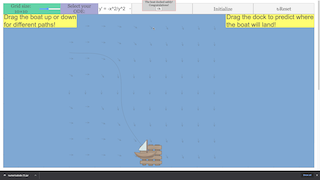Intro Page

 Following the Slope
 Let us change our focus from a differential equation that describes the motion of a meteor heading toward earth to the following differential equation: y'(x) = -x2/y2.     (4)
 For the sake of illustration, let us assume we cannot or do not want to solve this analytically (even though it is separable). Therefore, we do not know a function y(x) that satisfies (4). Again, if we did, such a function would be the analytic solution to (4). We do have information about the function, specifically the derivative of y for all points (x,y). For example, the derivative of y at the point (2,3) is -4/9. Recall that a derivative is the slope of the tangent line to the curve at that point. Therefore, let us draw an arrow with its tail at the point (2,3) and a slope of -4/9. What can this do for us? Let us repeat this process again for the points (1,2), (1,3), and (2,2) which produces the picture to the left below. We have produced a graph that is called a vector field or direction field. Whether this is helpful at this point may be a debatable topic. Let us repeat this process for more points which produces the vector field to the right below. Do you have a better sense of the direction of the underlying solutions?
 Now, rather than a vector field, let us imagine, as depicted below, that we are on the ocean and each vector in the graph gives the direction of the current at that point. Further, imagine that you can only discern the current's trajectory at those points where vectors are drawn. We will set sail and follow the underlying solution curve for that vector field. Can we sail our ship safely to the dock? To begin, we will have only a few vectors in the field as seen below to the left. In the Java application, you will be able to increase the number of vectors in the field. Will this help? Why or why not? Let us sail into the next exercise and explore this idea interactively

### Translations

Code Language Translator Run### Software Requirements

SoftwareRequirements

 Android iOS Windows MacOS with best with Chrome Chrome Chrome Chrome support full-screen? Yes. Chrome/Opera No. Firefox/ Samsung Internet Not yet Yes Yes cannot work on some mobile browser that don't understand JavaScript such as..... cannot work on Internet Explorer 9 and below

### CreditsWei Chiong Tan; Francisco Esquembre; Felix J. Garcia Clemente; Loo Kang WEE; Tim Chartier; Nicholas Dovidio

[text]

[text]

Research

[text]

[text]

[text]